Mathematical and Physical Journal
for High Schools
Issued by the MATFUND Foundation
 Already signed up? New to KöMaL?

# KöMaL Problems in Physics, February 2020

Show/hide problems of signs:## Problems with sign 'M'

Deadline expired on March 10, 2020.

M. 393. Hang a piece of twisted rope or yarn, and then attach different weights to its free end. Leave the rope unwind, continuously slowing the motion with your hand until it reaches the equilibrium position. How does the angle turned by the lower end of the rope depend on the load?

(6 pont)

statistics## Problems with sign 'G'

Deadline expired on March 10, 2020.

G. 697. We look into a kaleidoscope; part of the observed view is shown in the figure. Where are the mirrors of the kaleidoscope?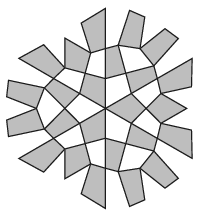(3 pont)

solution (in Hungarian), statistics

G. 698. We have three solid cubes of edges 1 cm, 3 cm and 9 cm. A tower is built from the three cubes. By what factor will the pressure exerted by the tower on the horizontal tabletop be greater when the smallest cube is at the bottom compared to when the largest cube is at the bottom?

(3 pont)

solution (in Hungarian), statistics

G. 699. At railway stations, we can often observe that the overhead contact wires are stretched with heavy weights suspended by wire ropes looped over, for example, a pulley system shown in the figure.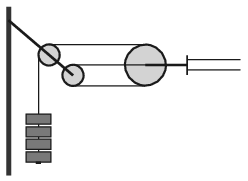$\displaystyle a)$ Why is this method better than fixing the overhead contact lines?

$\displaystyle b)$ What is the tension in each of the double overhead contact wires if the total mass of the weights is 300 kg?

$\displaystyle c)$ How does the position of the weights change during a bright cloudless day from dawn to dusk?

(4 pont)

solution (in Hungarian), statistics

G. 700. The 315 billion-tonne D28 iceberg (also known as Loose tooth, or Molar Berg) broke off Antarctica on 25 September 2019. If it was smashed to small ice-cubes and put into water at a temperature of $\displaystyle 20\;{}^\circ$C, how many times of the amount of the water in lake Balaton could be cooled down to a temperature of $\displaystyle 0\;{}^\circ$C? The volume of the water in lake Balaton is 1.9 km$\displaystyle {}^3$. Suppose that the temperature of the ice-berg is $\displaystyle -10\;{}^\circ$C everywhere.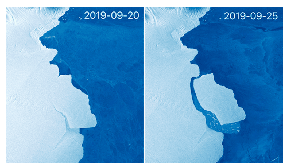(4 pont)

solution (in Hungarian), statistics## Problems with sign 'P'

Deadline expired on March 10, 2020.

P. 5197. As a present, Winnie-the-Pooh was given a sphere-shaped balloon of radius 20 cm. The balloon was filled with helium such that when Winnie-the-Pooh released its thread, it just floated in the air, so it neither rose up nor sank.

Winnie-the-Pooh was so happy that he began to scamper around in a circle with the balloon such that he held the end of the thread of the balloon in his hand. The balloon executed uniform circular motion. Piglet observed that whatever Winnie-the Pooh's constant angular speed was the thread of the balloon always had an angle of $\displaystyle 45^\circ$ with the tangent of the circular path of the balloon.

What was the radius of the path of the balloon? (Neglect the weight of the thread and the incidental changes in the shape of the balloon.)

(5 pont)

solution (in Hungarian), statistics

P. 5198. There is a prism-shaped object of mass $\displaystyle M$ on a horizontal smooth surface. One end of a light spring of spring constant $\displaystyle D$ is attached to the prism such that the spring is parallel to the symmetry axis of the prism, whilst the other end is fixed to a disc-shaped bumper of negligible mass. Another object of mass $\displaystyle m$, sliding at a speed of $\displaystyle v_0$, collides with the bumper such that it partly compresses the long enough spring.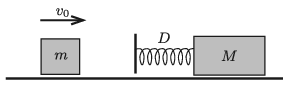$\displaystyle a)$ What is the speed of the centre of mass of the system?

$\displaystyle b)$ Starting the stopwatch at the moment when the object touches the bumper first, how much time elapses until the spring becomes the shortest?

(5 pont)

solution (in Hungarian), statistics

P. 5199. A piece of thin and rigid metal wire of length $\displaystyle \ell$ has a shape of a circular arc. Each end of the wire is attached to a thin thread of length $\displaystyle \ell$ and the other ends of the threads are fixed at the centre of the circular arc, $\displaystyle O$, as shown in the figure. The period of the pendulum, which can swing with small amplitude in the vertical plane of the figure, is $\displaystyle T_1$. If the metal wire is straightened then the pendulum with the altered shape has a period of $\displaystyle T_2$, when it swings about $\displaystyle O$ in the plane of the figure, with small amplitude. What is the ratio $\displaystyle T_2/ T_1$?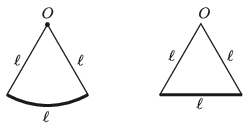(5 pont)

solution (in Hungarian), statistics

P. 5200. The speed of transverse waves in a suspension bridge over a valley is 400 m/s. In a storm the strong wind generated impulses, which are repeated in each second. What is the distance between the pillars if the bridge started to swing heavily?

(3 pont)

solution (in Hungarian), statistics

P. 5201. The figure shows a piston and two springs attached to it. The spring constant of both springs is $\displaystyle D=1000$ N/m, the ambient air pressure is $\displaystyle p_0=10^5$ Pa, and the piston of cross-sectional area $\displaystyle A=10~{\rm dm}^2$ encloses a sample of monatomic gas. Initially both springs are unstretched, and the volume of the gas is $\displaystyle V_0=50$ litres. How much does the piston move, if $\displaystyle Q=2~\rm kJ$ thermal energy is added to the sample of gas? (The walls of the container and the piston are thermally insulated; friction, and the heat capacity of the heating element are negligible.)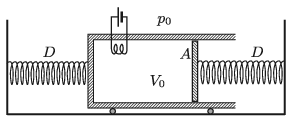(4 pont)

solution (in Hungarian), statistics

P. 5202. The specific heat capacity of metals at very low temperatures is approximately proportional to the absolute temperature ($\displaystyle c=\alpha \cdot T$, where the proportionality constant $\displaystyle \alpha$ is characteristic of the material). In a very well insulated chamber of a cryogenic laboratory, two pieces of different metals of different mass are placed such that they came into contact. The initial temperature of one of them (denoted by $\displaystyle A$) is 1.0 K, whilst that of the other $\displaystyle (B)$ is 3.0 K, and the final common temperature is 2.0 K. What will the final common temperature be if the initial temperature values of the metals are $\displaystyle T_A=1.5$ K and $\displaystyle T_B=2.5$ K?

(5 pont)

solution (in Hungarian), statistics

P. 5203. The refractive index of a transparent glass sheet of width $\displaystyle 2A$ varies in the direction of axis $\displaystyle z$, which is perpendicular to the plane of the sheet. At $\displaystyle z=\pm A$ its value is $\displaystyle n_0$, whilst at $\displaystyle z=0$ it is $\displaystyle n_1$. A thin ray of laser enters into the glass (at a height of'' $\displaystyle z= A$) and travels in the direction of axis $\displaystyle x$. The laser beam is deflected in the glass and travels along the curved path of a cosine function.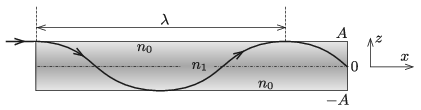$\displaystyle a)$ How does the refractive index depend on $\displaystyle z$?

$\displaystyle b)$ What is the wavelength of the path of the laser?

Data: $\displaystyle A=1$ cm, $\displaystyle n_0=1.5$ and $\displaystyle n_1=1.6$.

(5 pont)

solution (in Hungarian), statistics

P. 5204. Determine the sensitivity of the cathode-ray oscilloscope shown in the figure in the unit of mm/volt.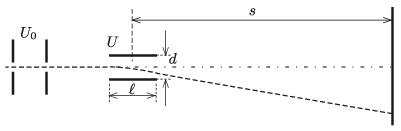Data: the length of the deflecting plates is $\displaystyle \ell=2$ cm, their distance is $\displaystyle d= 0.5$ cm, the distance between the screen and the centre of the plates is $\displaystyle s=20$ cm, the accelerating voltage is $\displaystyle U_0=1000$ V, and the greatest value of the deflecting voltage is $\displaystyle U_\text{max}=100$ V.

(4 pont)

solution (in Hungarian), statistics

P. 5205. The figure shows a loop made of a piece of copper wire. The shape of the loop is two concentric semi-circles and two connecting straight line segments. The loop is on a horizontal tabletop, but initially the smaller semi-circle is in a vertical position. The small semi-circle is turned into the horizontal position in 1 s. The dashed line is the axis of rotation. The whole loop is in uniform vertically upward magnetic field.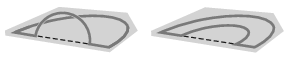$\displaystyle a)$ In which case is the flux linkage of the loop greater?

$\displaystyle b)$ What is the average value, and the direction of the induced current in the loop, while the smaller loop turns? What is the direction of the current?

$\displaystyle c)$ What is the greatest value of the induced current if the small semicircle is rotated at a constant angular speed and it takes exactly $\displaystyle \Delta t=1$ s to turn from the vertical position to the horizontal position?

Data: the magnetic induction is $\displaystyle B=0.35~\rm T$, the resistance of the loop is $\displaystyle R=0.025~\Omega$, the radius of the smaller semi-circle is $\displaystyle r=0.2$ m.

(4 pont)

solution (in Hungarian), statistics

P. 5206. Determine the atomic mass number of ionium, which is the daughter element of uranium, after the uranium emits two $\displaystyle \alpha$ and two $\displaystyle \beta$ particles. Which is the element whose isotope is the ionium?

(4 pont)

solution (in Hungarian), statistics

P. 5207. Muon ($\displaystyle \mu^-$) is an unstable elementary particle, its mean lifetime is 2.197 $\displaystyle \mu$s, its mass is 207 times the mass of an electron, and its charge is the same as the charge of an electron.

In a storage ring (a type of circular particle accelerator) there is uniform magnetic field, which is perpendicular to the plane of the ring. At a certain point of the ring, from the direction of the tangent at that point, a mono-energetic muon beam is injected into the storage ring. The muons revolve along the circular path and on average they decay after completing five whole turns.

$\displaystyle a)$ What is the (average) speed and kinetic energy of the muons if the radius of the storage ring is 120 m?

$\displaystyle b)$ What is the magnetic induction in the storage ring?

(6 pont)

solution (in Hungarian), statistics